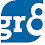# Line Graphs

The line graph is another important type of graph. The line graph displays information as a series of data points connected by some straight line segments (or simply lines). You can simply say that the Line Graph is the graph which uses points connected by lines to show how something changes in value. Lets have a look at an example Line Graph so that we can understand the topic in detail.

Single Line Graph :

The following Line Graph gives the production of a company over the years 1999 to 2005.

Now the problem question will be like this. In how many of the following years was the production of the company above the average? (followed by some options)

Sol :

The Average Production =
So clearly it is visible that the production is above average in 3 years.

Multiple Line Graph :

The following Graph shows the profit of two companies A and B (In Lakhs) over the years 2000 to 2005.
Now the question will be like this. What is the difference between the average profit of the companies A and B over the years? (followed by some options)

Sol :
So 3.33 Lakhs is the answer :)

1.Pls give me Solved Problem of Statistics in Ratio type which is frequently asked in po exam of 2012 i.e 5:6 A&B.

2.please give some tough problems DI is really tough in the exam

3.Sir this problems are simple but we r getting very very difficult problems in po this like problems of IIM.

1.This is just a model problem Aruna. We will provide you with the problems of Higher difficulty level soon. Thank you so much for the feedback.